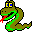#Boost C++ Libraries

...one of the most highly regarded and expertly designed C++ library projects in the world.

This is the documentation for an old version of Boost. Click here to view this page for the latest version.#kruskal_minimum_spanning_tree

```template <class Graph, class OutputIterator, class P, class T, class R>
OutputIterator
kruskal_minimum_spanning_tree(Graph& g, OutputIterator tree_edges,
const bgl_named_params<P, T, R>& params = all defaults);
```

The kruskal_minimum_spanning_tree() function find a minimum spanning tree (MST) in an undirected graph with weighted edges. A MST is a set of edges that connects all the vertices in the graph where the total weight of the edges in the tree is minimized. For more details, see section Minimum Spanning Tree Problem. The edges in the MST are output to the tree_edges output iterator. This function uses Kruskal's algorithm to compute the MST [18,8,27,15].

Kruskal's algorithm starts with each vertex in a tree by itself, and with no edges in the minimum spanning tree T. The algorithm then examines each edge in the graph in order of increasing edge weight. If an edge connects two vertices in different trees the algorithm merges the two trees into a single tree and adds the edge to T. We use the ``union by rank'' and ``path compression'' heuristics to provide fast implementations of the disjoint set operations (MAKE-SET, FIND-SET, and UNION-SET). The algorithm is as follows:

```KRUSKAL-MST(G, w)
T := Ø
for each vertex u in V
MAKE-SET(DS, u)
end for
for each edge (u,v) in E in order of nondecreasing weight
if FIND-SET(DS, u) != FIND-SET(DS, v)
UNION-SET(DS, u, v)
T := T U {(u,v)}
end for
return T
```

### Parameters

IN: const Graph& g
An undirected graph. The graph type must be a model of Vertex List Graph and Edge List Graph.
Python: The parameter is named graph.
IN: OutputIterator spanning_tree_edges
The edges of the minimum spanning tree are output to this Output Iterator.
Python: This parameter is not used in Python. Instead, a Python list containing all of the spanning tree edges is returned.

### Named Parameters

IN: weight_map(WeightMap w_map)
The weight or ``length'' of each edge in the graph. The WeightMap type must be a model of Readable Property Map and its value type must be Less Than Comparable. The key type of this map needs to be the graph's edge descriptor type.
Default: get(edge_weight, g)
Python: Must be an edge_double_map for the graph.
Python default: graph.get_edge_double_map("weight")
UTIL: rank_map(RankMap r_map)
This is used by the disjoint sets data structure. The type RankMap must be a model of Read/Write Property Map. The vertex descriptor type of the graph needs to be usable as the key type of the rank map. The value type of the rank map must be an integer type.
Default: an iterator_property_map created from a std::vector of the integers of size num_vertices(g) and using the i_map for the index map.
Python: Unsupported parameter.
UTIL: predecessor_map(PredecessorMap p_map)
This is used by the disjoint sets data structure, and is not used for storing predecessors in the spanning tree. The predecessors of the spanning tree can be obtained from the spanning tree edges output. The type PredecessorMap must be a model of Read/Write Property Map. The key type value types of the predecessor map must be the vertex descriptor type of the graph.
Default: an iterator_property_map created from a std::vector of vertex descriptors of size num_vertices(g) and using the i_map for the index map.
Python: Unsupported parameter.
IN: vertex_index_map(VertexIndexMap i_map)
This maps each vertex to an integer in the range [0, num_vertices(g)). This is only necessary if the default is used for the rank or predecessor maps. The type VertexIndexMap must be a model of Readable Property Map. The value type of the map must be an integer type. The vertex descriptor type of the graph needs to be usable as the key type of the map.
Default: get(vertex_index, g) Note: if you use this default, make sure your graph has an internal vertex_index property. For example, adjacenty_list with VertexList=listS does not have an internal vertex_index property.
Python: Unsupported parameter.

### Complexity

The time complexity is O(E log E)

### Example

The file examples/kruskal-example.cpp contains an example of using Kruskal's algorithm.

 Copyright © 2000-2001 Jeremy Siek, Indiana University (jsiek@osl.iu.edu)